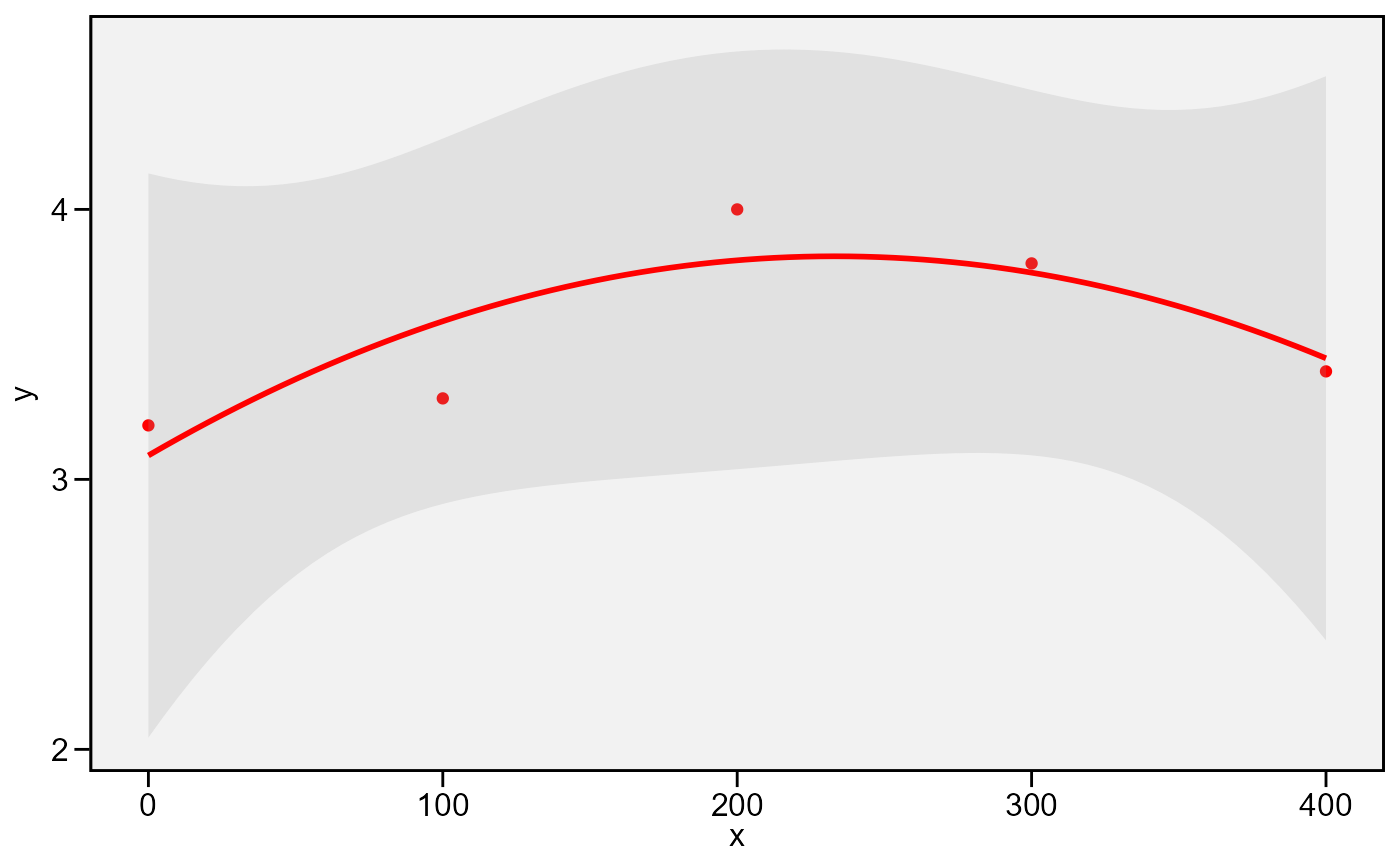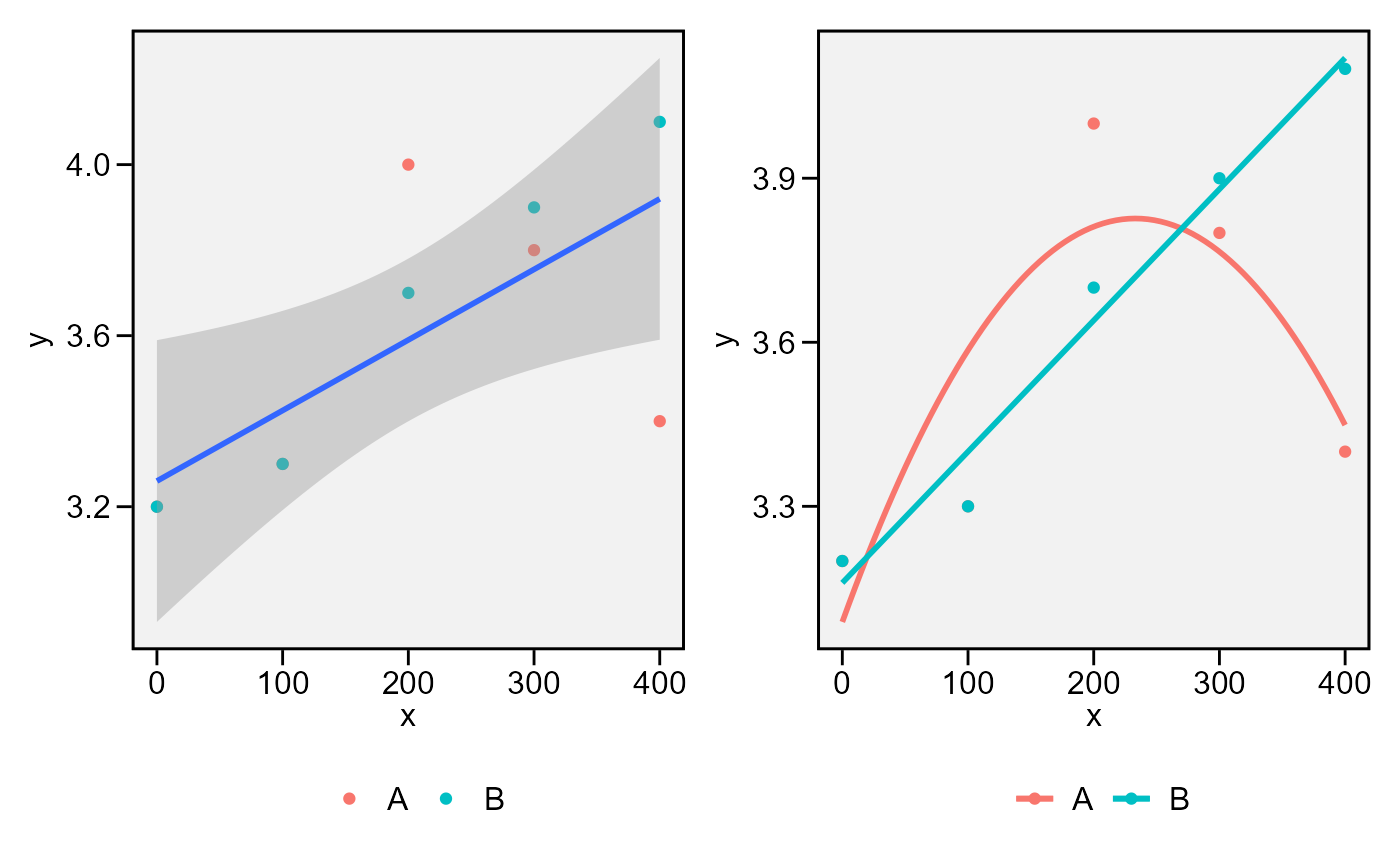• plot_lines() Creates a line plot based on one quantitative factor and one numeric variable. It can be used to show the results of a one-way trial with quantitative treatments.

• plot_factlines() Creates a line plot based on: one categorical and one quantitative factor and one numeric variable. It can be used to show the results of a two-way trial with qualitative-quantitative treatment structure.

## Usage

plot_lines(
.data,
x,
y,
fit,
level = 0.95,
confidence = TRUE,
xlab = NULL,
ylab = NULL,
n.dodge = 1,
check.overlap = FALSE,
col = "red",
alpha = 0.2,
size.shape = 1.5,
size.line = 1,
size.text = 12,
fontfam = "sans",
plot_theme = theme_metan()
)

plot_factlines(
.data,
x,
y,
group,
fit,
level = 0.95,
confidence = TRUE,
xlab = NULL,
ylab = NULL,
n.dodge = 1,
check.overlap = FALSE,
legend.position = "bottom",
grid = FALSE,
scales = "free",
col = TRUE,
alpha = 0.2,
size.shape = 1.5,
size.line = 1,
size.text = 12,
fontfam = "sans",
plot_theme = theme_metan()
)

## Arguments

.data

The data set

x, y

The variables to be mapped to the x and y axes, respectively.

fit

The polynomial degree to use. It must be between 1 (linear fit) to 4 (fourth-order polynomial regression.). In plot_factlines(), if fit is a lenth 1 vector, i.e., 1, the fitted curves of all levels in group will be fitted with polynomial degree fit. To use a different polynomial degree for each level in group, use a numeric vector with the same length of the variable in group.

level

The fonfidence level. Defaults to 0.05.

confidence

Display confidence interval around smooth? (TRUE by default)

xlab, ylab

The labels of the axes x and y, respectively. Defaults to NULL.

n.dodge

The number of rows that should be used to render the x labels. This is useful for displaying labels that would otherwise overlap.

check.overlap

Silently remove overlapping labels, (recursively) prioritizing the first, last, and middle labels.

col

The colour to be used in the line plot and points.

alpha

The alpha for the color in confidence band

size.shape

The size for the shape in plot

size.line

The size for the line in the plot

size.text

The size of the text

fontfam

The family of the font text.

plot_theme

The graphical theme of the plot. Default is plot_theme = theme_metan(). For more details, see ggplot2::theme().

group

The grouping variable. Valid for plot_factlines() only.

legend.position

Valid argument for plot_factlines. The position of the legend. Defaults to 'bottom'.

grid

Valid argument for plot_factlines. Logical argument. If TRUE then a grid will be created.

scales

Valid argument for plot_factlines. If grid = TRUE scales controls how the scales are in the plot. Possible values are 'free' (default), 'fixed', 'free_x' or 'free_y'.

## Value

An object of class gg, ggplot.

plot_bars() and plot_factbars()

## Author

Tiago Olivoto tiagoolivoto@gmail.com

## Examples

# \donttest{
library(metan)
# One-way line plot
df1 <- data.frame(group = "A",
x = c(0, 100, 200, 300, 400),
y = c(3.2, 3.3, 4.0, 3.8, 3.4))
plot_lines(df1, x, y, fit = 2)# Two-way line plot
df2 <- data.frame(group = "B",
x = c(0, 100, 200, 300, 400),
y = c(3.2, 3.3, 3.7, 3.9, 4.1))
facts <- rbind(df1, df2)

p1 <- plot_factlines(facts, x, y, group = group, fit = 1)
p2 <- plot_factlines(facts,
x = x,
y = y,
group = group,
fit = c(2, 1),
confidence = FALSE)
arrange_ggplot(p1, p2)# }